Courses
Courses for Kids
Free study material
Offline Centres
More

# Bernoulli’s PrincipleLast updated date: 02nd Dec 2023
Total views: 405.9k
Views today: 6.05k## An Introduction to Bernoulli’s Theorem

Bernoulli’s theorem is the principle of energy conservation for perfect fluids in steady or streamlined flow. The fluid dynamics discussed by Bernoulli's theorem include how the fluid pressure varies with the flow velocity. The theorem was developed by Daniel Bernoulli, a Swiss mathematician in 1738. Leonard Euler created the Bernoulli equation that is still in use today despite Daniel Bernoulli having identified the underlying idea.

## History of Daniel Bernoulli

Daniel Bernoulli (1700–1782) was a Dutch scientist who studied in Italy before settling in Switzerland. He was born into a family of mathematicians, his father, Johann Bernoulli, was one of the early pioneers of calculus, and his uncle, Jacob Bernoulli, was the first to discover probability theory.Daniel Bernoulli (1700–1782),

## Bernoulli's Principle

Bernoulli’s theorem, also known as Bernoulli’s principle, states that the whole mechanical energy of the moving fluid, which includes gravitational potential energy of elevation, fluid pressure energy, and kinetic energy of fluid motion, remains constant.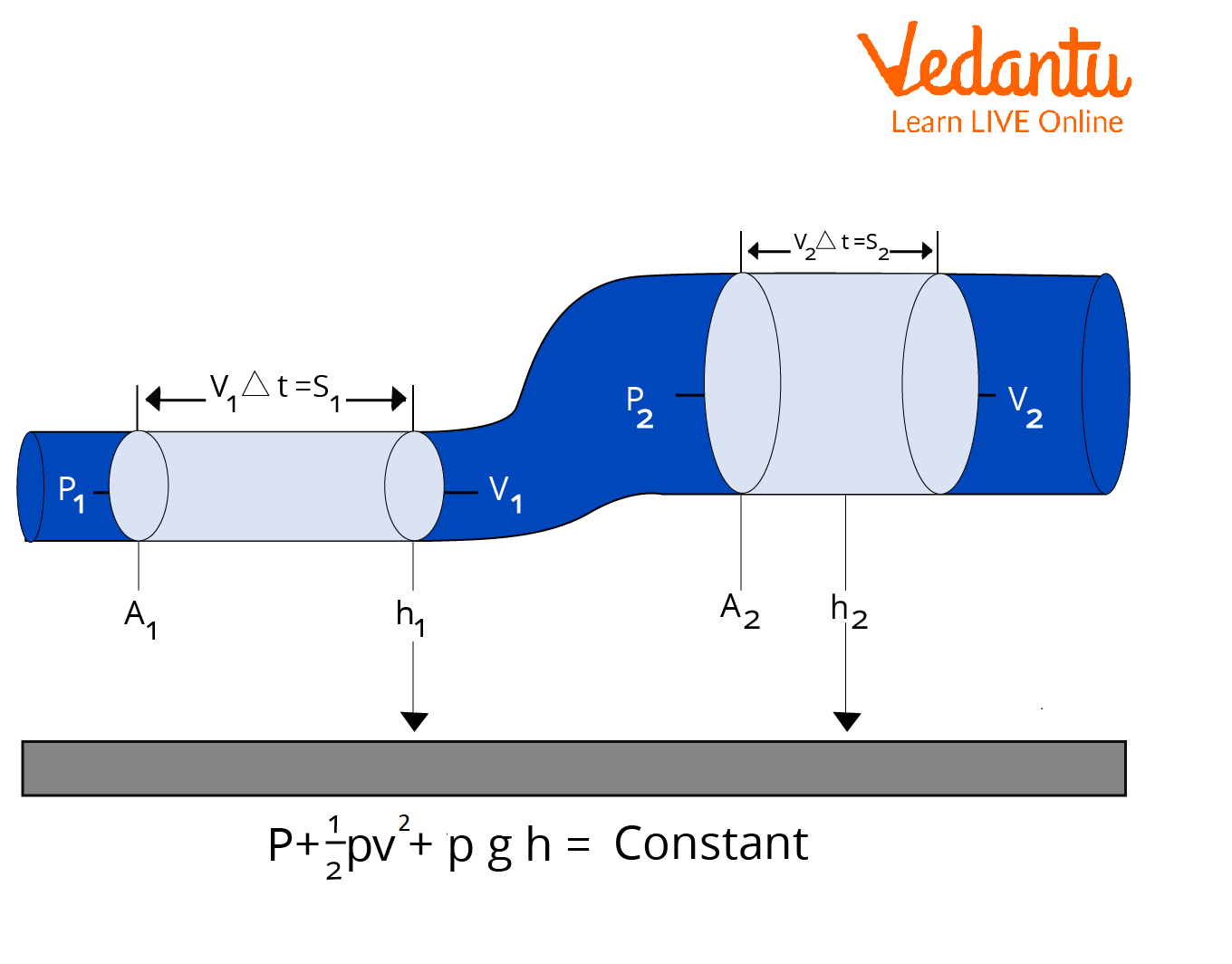Bernoulli Equation

## Assumption of Bernoulli's Theorem

The assumptions made to derive Bernoulli’s equation are as follows:

• The fluid is excellent or perfect, with zero viscosity.

• The flow is constant.

• While flowing, there is no energy loss.

• It is not possible to compress the flow.

• Irrotational flow is present.

• Except for gravity, there is no external force acting on the liquid.

## Limitations of Bernoulli’s Theorem

• The velocity of each liquid component moving through each cross-section of the pipe has been assumed to be uniform to derive the Bernoulli equation. It is not true actually.

• This formula is ineffectual in turbulent or non-steady flows.

• This theorem is typically used for low viscosity fluids.

## Application of Bernoulli Theorem

Some of the important applications of Bernoulli’s theorem are given below:

• One of Bernoulli's theorem applications is that it is used to solve any problems involving incompressible fluid flow.

• Venturimeter is a tool that utilises Bernoulli's theorem to gauge the flow rate of the liquid via pipes. It is made up of a skinny coaxial tube B that joins two identical coaxial tubes A and C. To gauge the pressure of the next liquid, two vertical tubes D and E are installed on tubes A and B.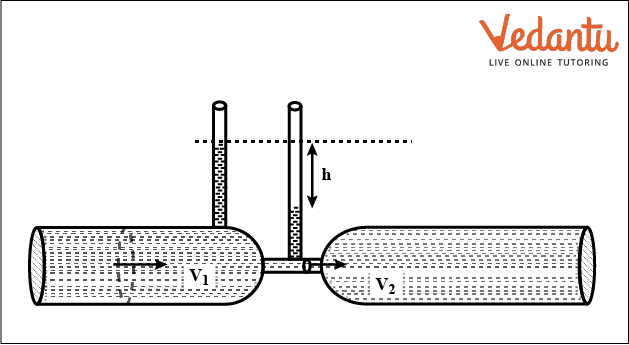Venturimeter

When a liquid flows through tube ABC, part B will experience a higher flow velocity than either tube A or C. Therefore, component B's pressure will be lower than that of tubes A or C. It is possible to determine the rate of flow of the liquid in the tube by measuring the pressure differential between A and B.

• An aircraft's airspeed can be calculated using its static port and Pitot tube. The airspeed indicator is calibrated using Bernoulli's principle to show the indicated airspeed that corresponds to the dynamic pressure.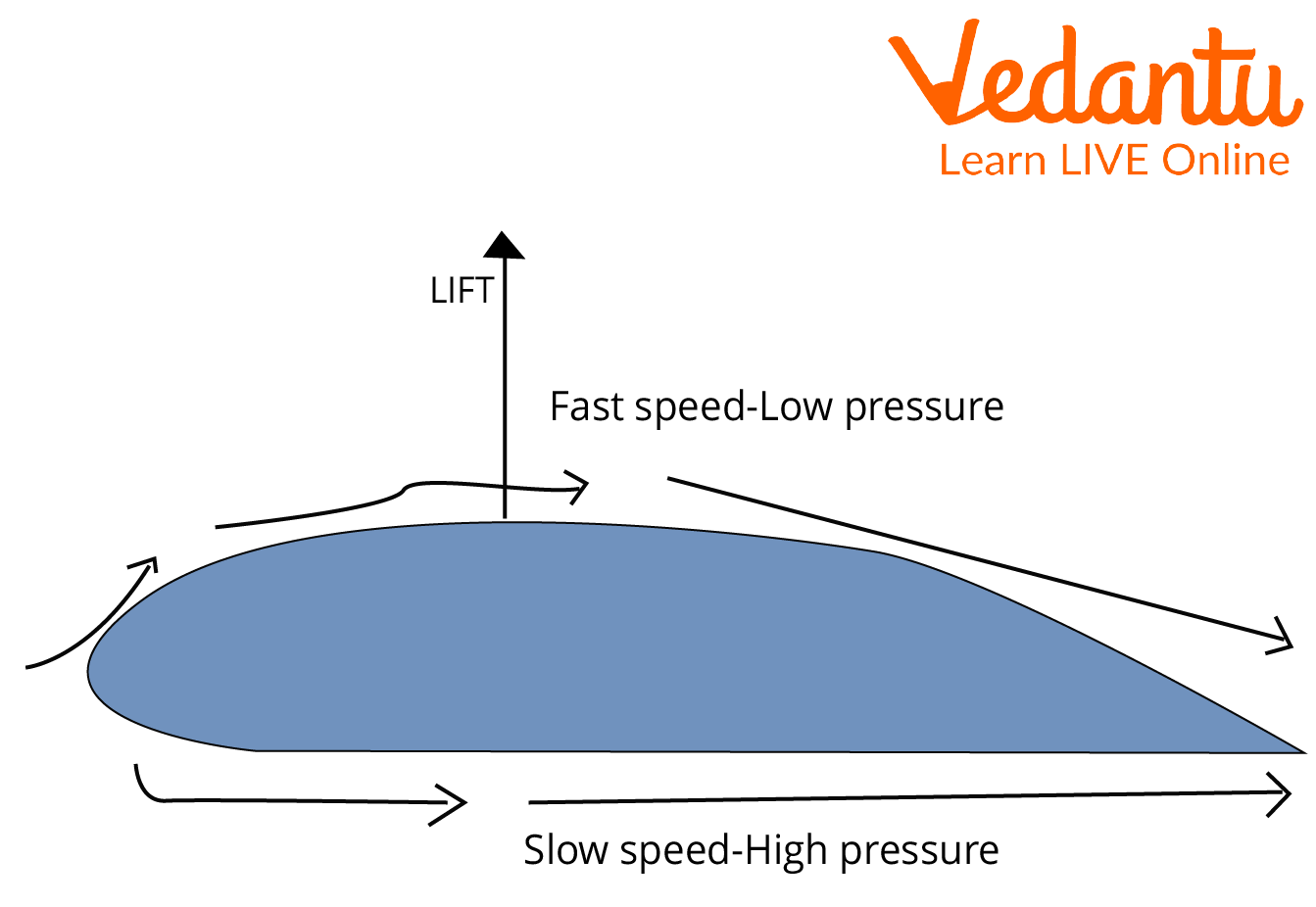Aeroplane’s Wing

• When using a bunsen burner, the gas leaves the nozzle quickly, which causes the pressure in the stem to drop. Consequently, fresh air from outside enters the burner through an air vent, and the resulting mixture of gas and air produces a blue flame.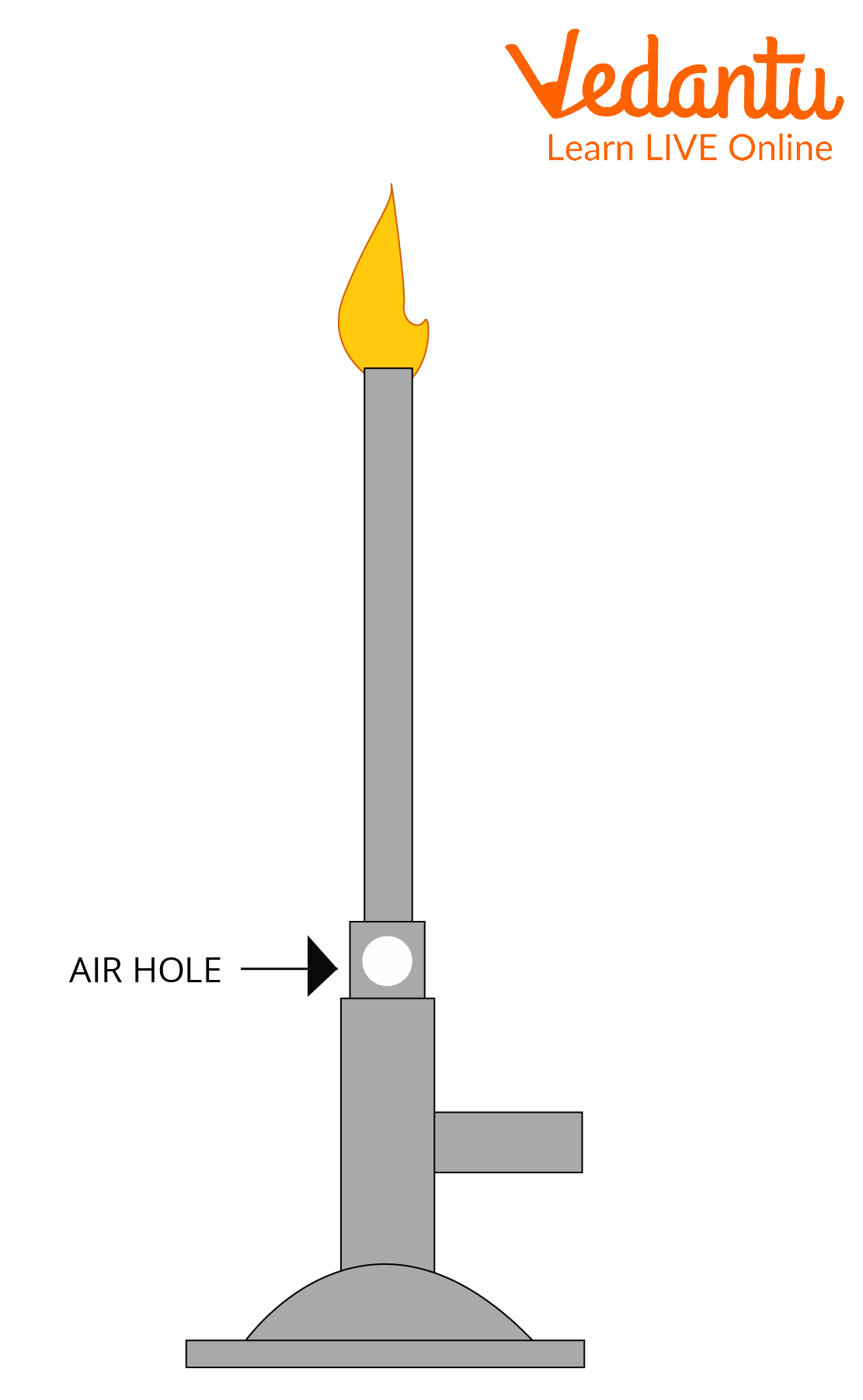Bunsen Burner

## Solved Examples

1. What are examples that use the Bernoulli principle?

1. Venturi metre

2. Mosquito Sprayers

3. Barometer

4. Thermometer

Ans. The following claims are based on Bernoulli's principle:

• Venturi metre

• Mosquito spray

• A barometer using hydrostatic pressure.

• Alcohol-based on expansion or a mercury thermometer

2. To have the most lift power possible, aircraft wings are made to seem like a picture. Utilise the Bernoulli theorem to explain why the design is created that way. ( given: v is the air flow's velocity and P is the air pressure).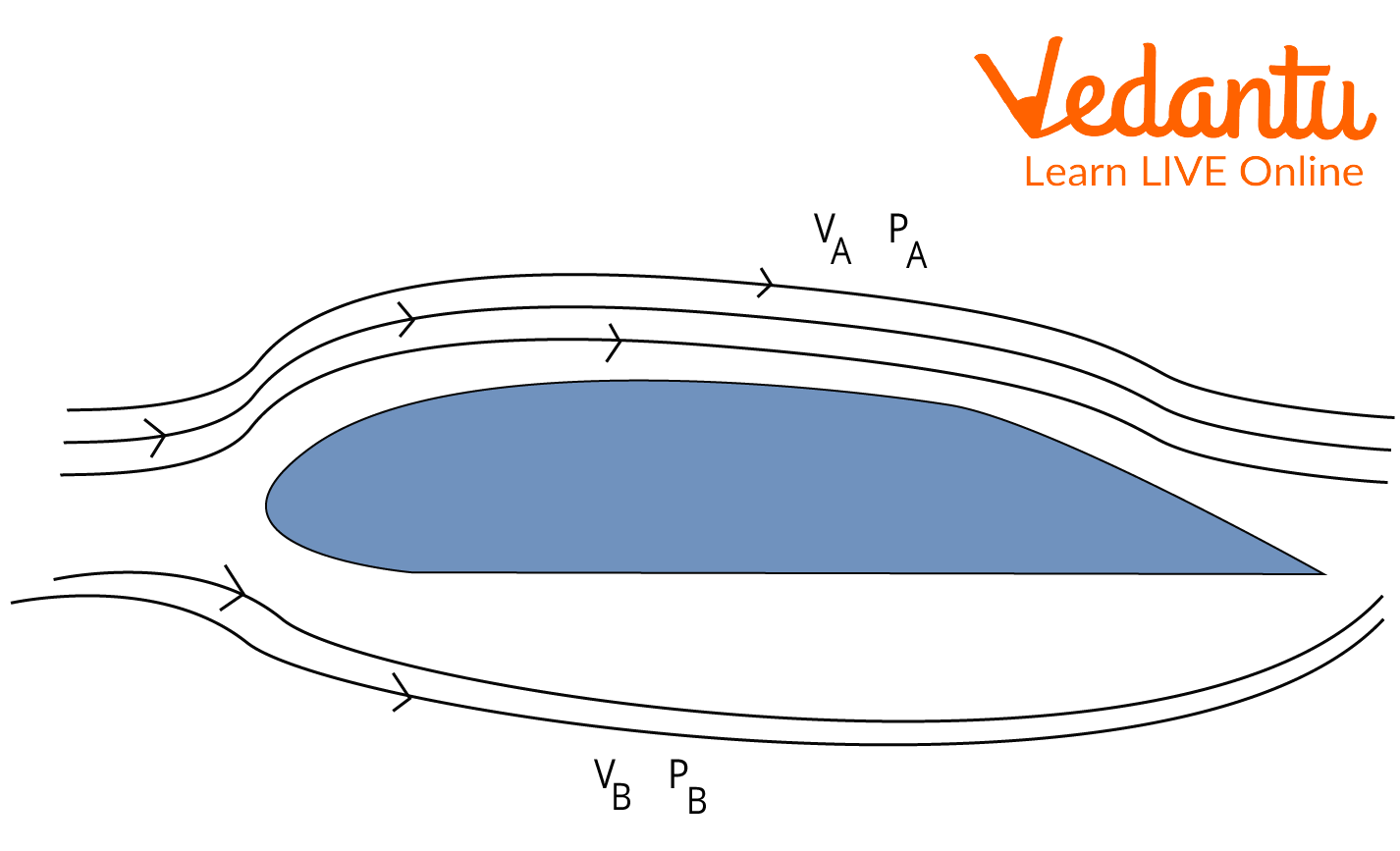Streamlined Body

Ans. It is given that v is the air flow's velocity and P is the air pressure.

The air thrust at the bottom of the wing must be greater than the thrust force at the top of the wing for the plane's wings to lift upward.

When the air pressure at the bottom of the wing is higher, there is a greater thrust force on the underside of the wing. The pressure of P is exactly proportional to F, and its equation is $P = F/A$. When the pressure is higher, the force also rises. According to Bernoulli's principle, when the air velocity near the bottom of the wing is low, the air pressure there is high.

$\begin{array}{l}{V_A} > {V_B}\\\therefore {P_A} < {P_B}\end{array}$

## Important Points

• This formula is only for perfect fluids in steady or streamlined flow.

• Bernoulli's theorem says, slower flowing fluids generate more pressure than faster moving fluids.

## Conclusion

Bernoulli's theorem explains the connection between pressure, velocity, and elevation in a flowing liquid or gas. The principle gives insight into a variety of applications. The best approach to grasp these various applications is to have an open debate about the idea itself.

## FAQs on Bernoulli’s Principle

1. Explain how, according to the Bernoulli Principle, slower flowing fluids generate more pressure (force) than faster moving fluids.

Bernoulli's principle, often known as Bernoulli's equation, will apply to ideal fluids. As a result, pressure and density are inversely proportional. That means that a slow-moving fluid will exert more pressure than faster-moving fluid. The discovery of this concept was made by Swiss scientist Daniel Bernoulli while testing with fluid inside pipes. His experiment discovered that as the fluid's speed increases, its internal pressure falls. Therefore, according to this principle, slower flowing fluids generate more pressure (force) than faster moving fluids.

2. How does Bernoulli's equation make it possible to guess how quickly and how forcefully fluid is flowing through a pipe?

Bernoulli's theorem provides a relationship between velocity and pressure. So It enables us to quantify the change in velocity and pressure that a fluid experiences when it travels from one pipe with one cross-section to another with a different cross-section. As a fluid moves from a larger conduit to a smaller pipe, its velocity and pressure rise. By maintaining constant fluid pressure, this relationship is particularly crucial in preventing a breakdown in water pipelines. The pipe explodes if the pressure is too great, resulting in damage and other issues.

3. What is Bernoulli's effect?

Air pressure in a moving fluid is reduced due to the Bernoulli effect. For instance, when air is blown over the strip of paper, which is held just below the lips, the static atmosphere is under a little bit less pressure than the stream of moving air above the paper. Due to its increased pressure, the static air beneath the paper is more likely to travel in that direction. The greater pressure of static air pushes the paper upward since it is blocking the way.